## 中国医科大学23春学期《系统解剖学(本科)》实践考试试题

------------------------
1.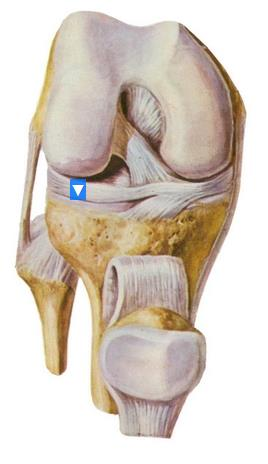A.内侧半月板
B.胫骨
C.腓骨
D.髌骨
E.外侧半月板

2.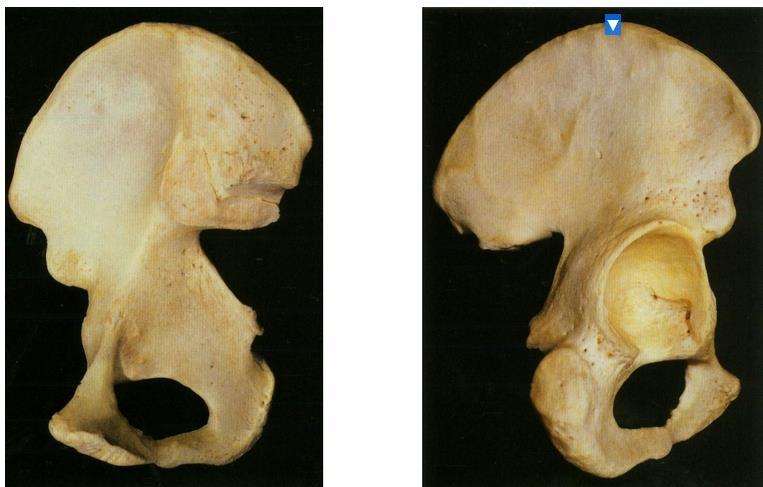A.髂棘
B.弓状线
C.髂前下棘
D.坐骨
E.耻骨结节

3.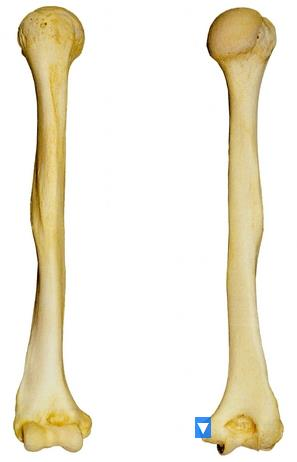A.大结节
B.内上髁
C.结节间沟
D.小结节
E.桡神经沟

4.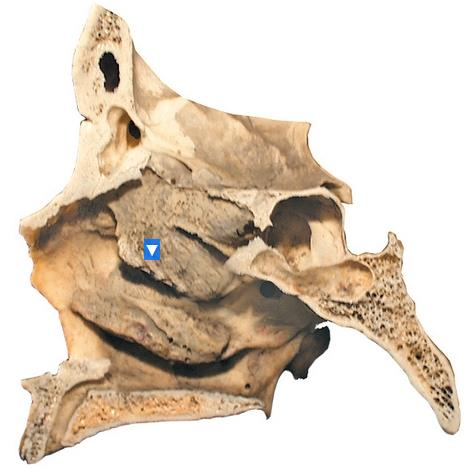A.额窦
B.上鼻甲
C.下鼻道
D.中鼻甲

5.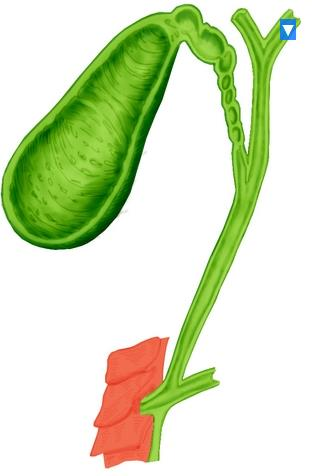A.肝右管
B.胆总管
C.胆囊管
D.肝左管
E.肝总管

6.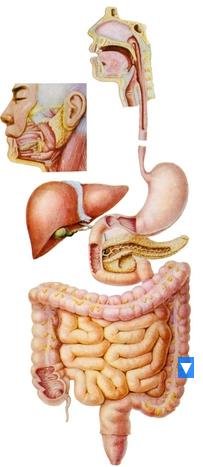A.乙状结肠
B.升结肠
C.阑尾
D.降结肠
E.横结肠

7.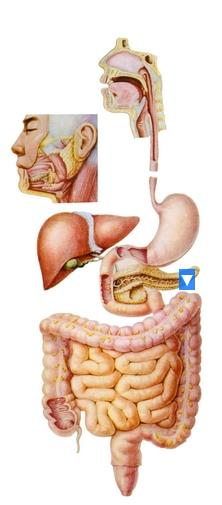A.肝
B.食管
C.十二指肠
D.胰
E.胃

8.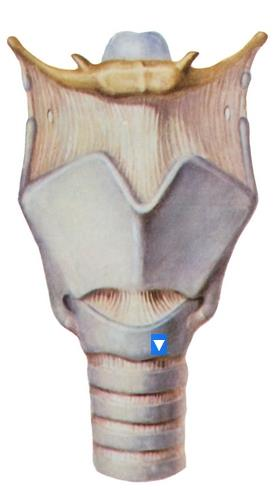A.会厌软骨
B.舌骨
C.甲状软骨
D.环状软骨弓
E.气管软骨

9.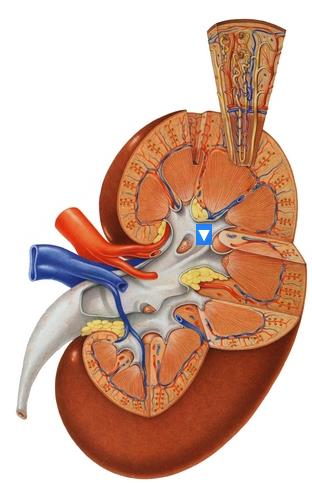A.肾锥体
B.肾大盏
C.肾乳头
D.肾盂
E.肾小盏

10.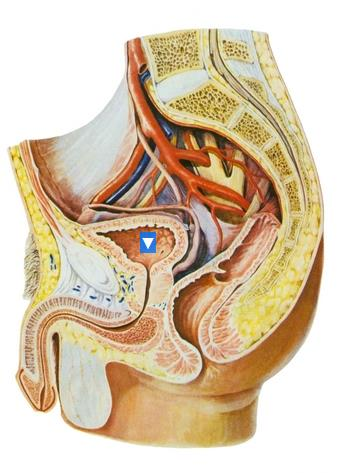A.直肠
B.尿道外口
C.耻骨联合
D.尿道膜部
E.膀胱

11.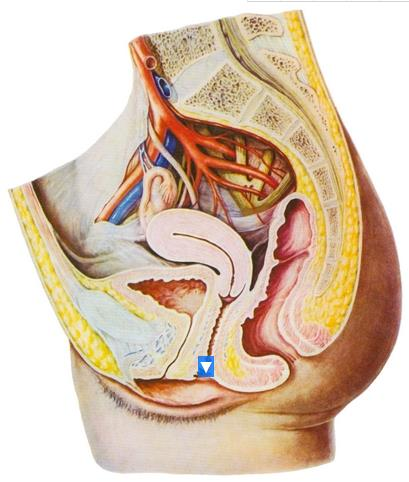A.直肠子宫陷凹
B.尿道
C.阴道穹(后部)
D.阴道
E.子宫

12.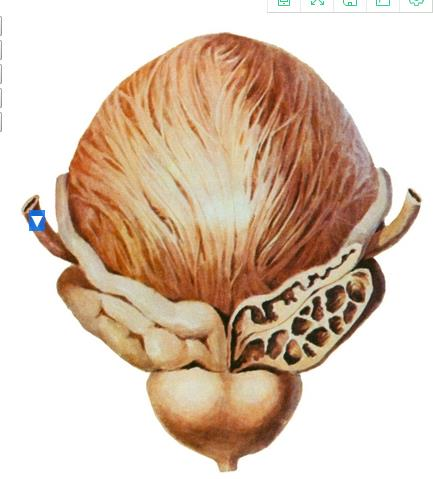A.前列腺
B.输精管壶腹
C.精囊
D.输精管
E.输尿管

13.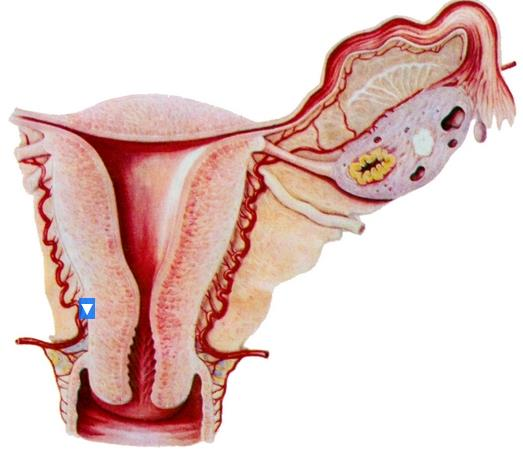A.子宫体
B.子宫峡
C.子宫腔
D.子宫颈
E.子宫底

14.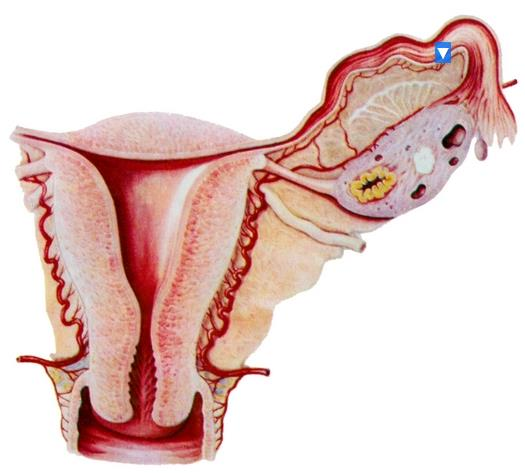A.卵巢
B.输卵管漏斗
C.卵巢固有韧带
D.输卵管壶腹
E.输卵管伞

15.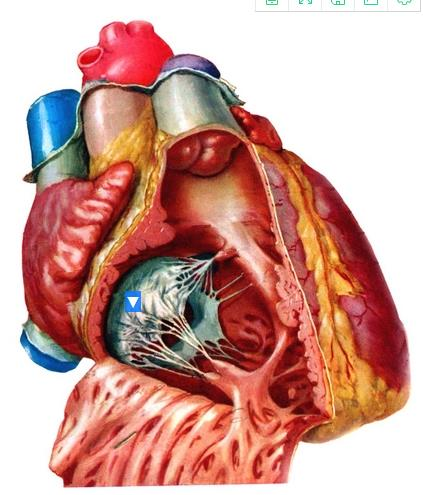A.右心耳
B.右房室瓣前尖
C.隔缘肉柱
D.乳头肌
E.腱索

16.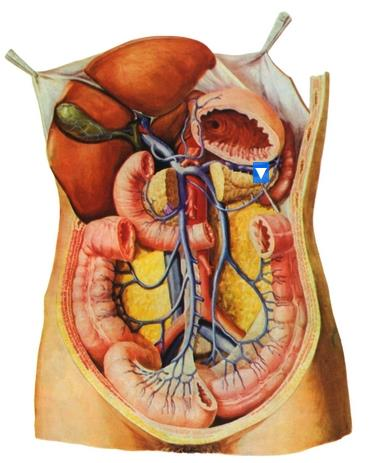A.肠系膜上静脉
B.肠系膜下静脉
C.肝门静脉
D.直肠上静脉
E.脾静脉

17.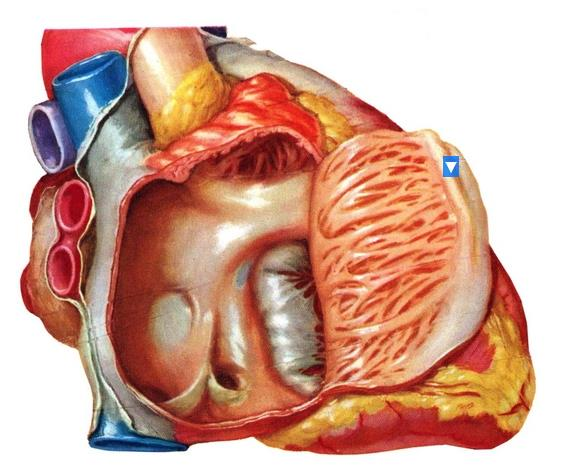A.右房室瓣
B.梳状肌
C.冠状窦口
D.卵圆窝
E.界嵴

18.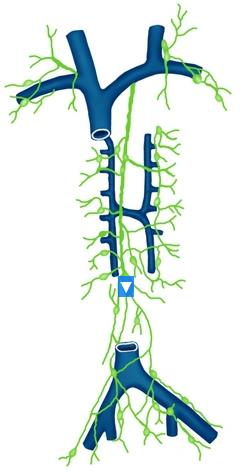A.奇静脉
B.左支气管纵隔干
C.左颈干
D.胸导管
E.乳糜池

19.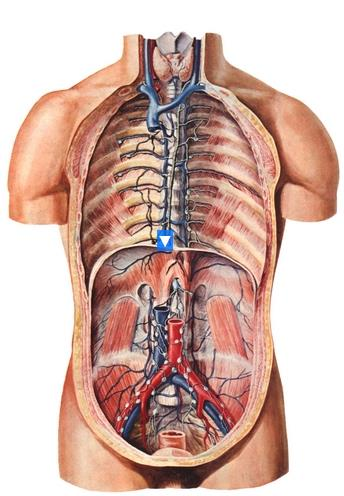A.奇静脉
B.胸导管
C.上腔静脉
D.半奇静脉
E.头臂静脉

20.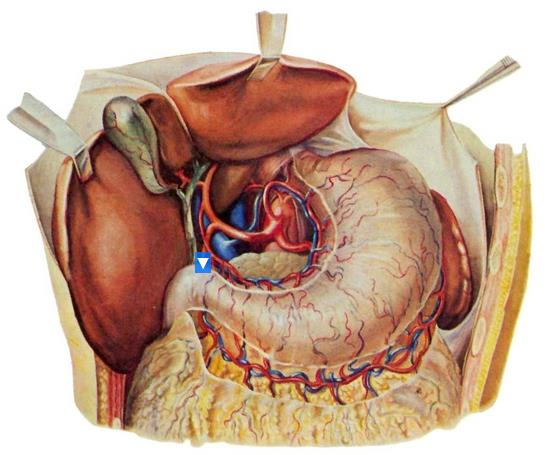A.胃十二指肠动脉
B.腹腔干
C.肝固有动脉
D.脾动脉

21.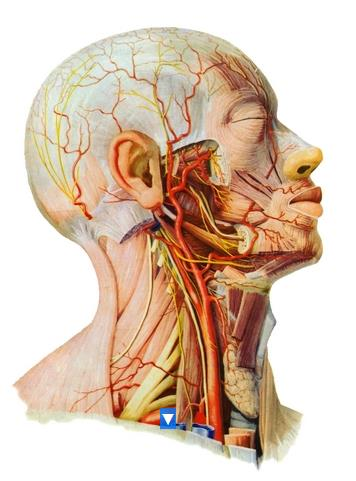A.颈总动脉
B.臂丛
C.颈外动脉
D.颈内动脉
E.锁骨下动脉

22.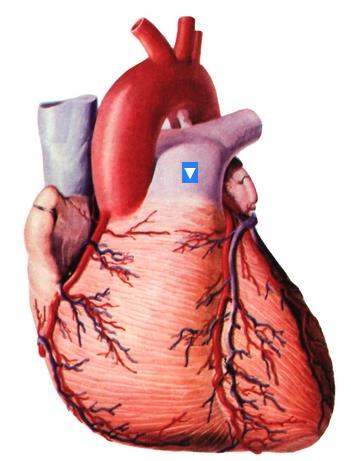A.肺动脉干
B.左颈总动脉
C.动脉韧带
D.左锁骨下动脉
E.头臂干

23.A.薄束结节
B.小脑中脚
C.楔束结节
D.滑车神经
E.松果体

24.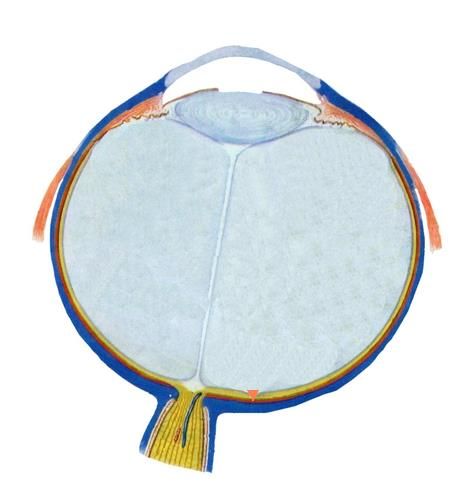A.视神经盘
B.视网膜
C.玻璃体
D.前房
E.中央凹

25.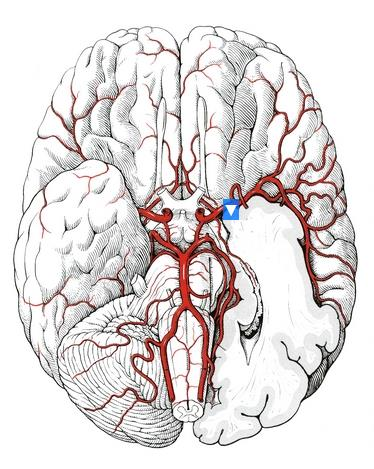A.椎动脉
B.大脑中动脉
C.大脑后动脉
D.后交通动脉
E.基底动脉

26.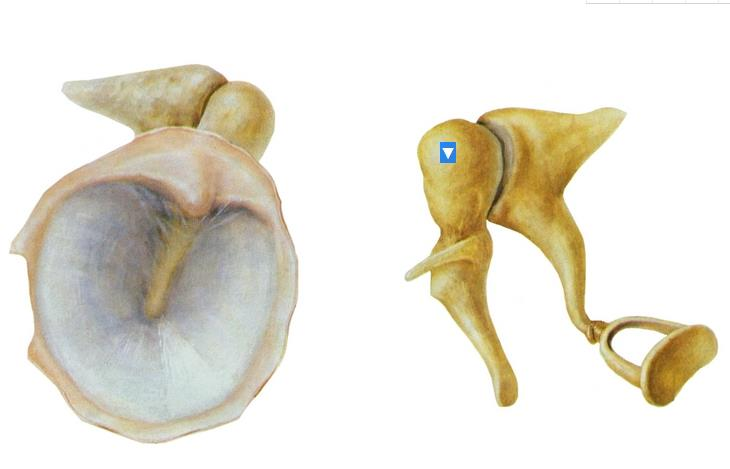A.镫骨
B.砧骨短脚
C.砧骨长脚
D.锤骨柄
E.锤骨头

27.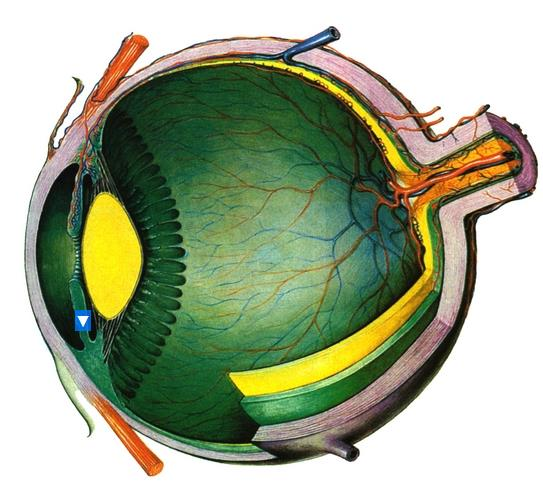A.虹膜
B.晶状体
C.角膜
D.睫状体
E.巩膜

28.A.第四脑室
B.胼胝体干
C.胼胝体膝
D.胼胝体压部
E.扣带回

29.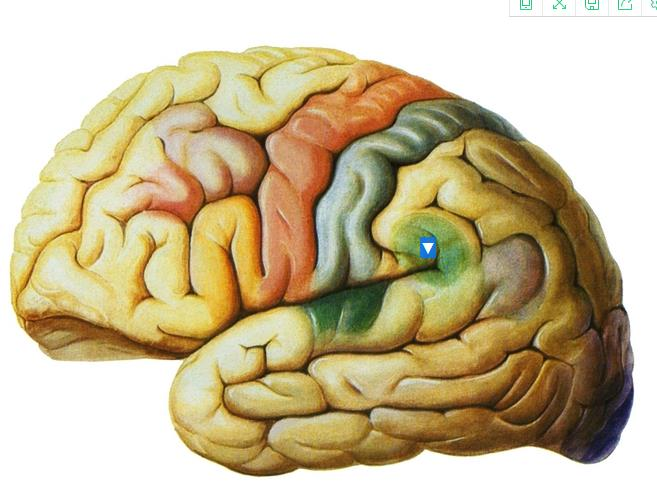A.中央前回
B.外侧沟
C.缘上回
D.中央后回
E.角回

30.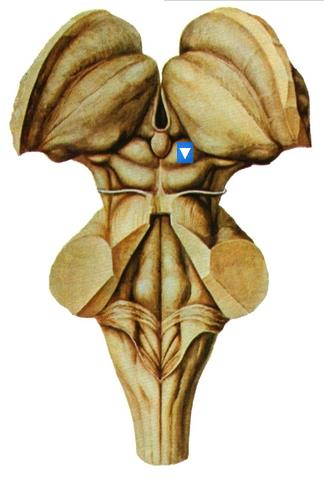A.面神经丘
B.背侧丘脑
C.下丘
D.上丘
E.尾状核